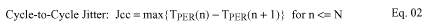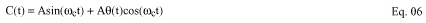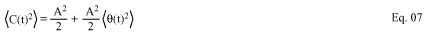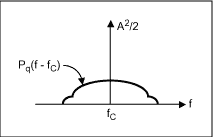# Jitter Measurements for CLK Generators or Synthesizers

### Abstract

Clock (CLK) generators and synthesizers form the pulse of a complex digital system and errors in a clock's signal quality can have wide-ranging effect.

One of the most important performance measurements is clock jitter. Jitter is defined as \"the short-term variation of a signal with respect to its ideal position in time.\" In a clock generator chip, there are many factors which contribute to output clock jitter, such as the device noise, supply variation, jitter in the reference clock, loading condition, and interference coupled from nearby circuitry.

Jitter can be measured in different ways, including period jitter JPER, cycle-to-cycle jitter JCC, and accumulated jitter JACC. In general, jitter consists of the deterministic jitter and random jitter. Since the deterministic jitter in most digital systems is introduced frommany different bit patterns from the digital data, clock signal jitter can usually be considered to be just random jitter. In this application note, we will go over the definitions and introduce test setups for making measurements.

### Period Jitter: JPER

Jitter JPER is the most popular jitter measure. It is the time difference between a measured cycle period and the ideal cycle period. Due to its random nature, this jitter can be measured as Peak-to-Peak or by Root Mean Square (RMS). Let's define the clock rising edge crossing point at the threshold VTH as TPER(n), where n is the time domain index, as shown in Figure 1. Mathematically, we can describe JPER aswhere T0 is the ideal clock cycle. Figure 1 shows the relation between JPER and TPER in a clock waveform.Figure 1. Period Jitter Measurement.

### Cycle to Cycle Jitter: Jcc

Jitter Jcc is the time difference of two adjacent clock periods. This is also called "Short Term Jitter." It depends only on the adjacent clock cycles.

For some digital circuits, Jcc is more meaningful than JPER. Jcc is the right parameter for calculating the margins for set-up time and hold-on time. If only JPER is employed in the calculation, the result is often too conservative. As an extreme example, to a spread spectrum (SS) clock, Jcc can be relatively small, but its JPER can be very large when the measuring time is comparable to the spreading period.

Jcc is measured by its Peak-to-Peak value in a given time period. It is mathematically defined aswhere n is a cycle index and N is a number for a given period. Figure 2 shows the relation between Jcc and TPER(n) in a clock waveform.Figure 2. Cycle-to-Cycle Jitter Measurement.

### Accumulated Jitter: JAC(n)

Jitter JAC(n) is the time displacement of the edges of a clock relative to the triggering edge of the same clock. This jitter is a function of n and it is the general case of JPER. It is measured by its Peak-to-Peak value or RMS. It gives the maximum discrepancy between two clock rising edges n cycles apart. For a digital system with synchronized packet data, this jitter measure may be the right one for system timing analysis. Its mathematic form is given asIt is noted that JAC(n) is dependent on the cycle index n. Figure 3 depicts how to measure JAC(n) from a clock signal.Figure 3. Accumulated jitter measurement.

### Time Domain Jitter Measurement

A high precision digital oscilloscope is commonly used to measure jitter. When the clock jitter is 10 times or larger than the triggering jitter of the oscilloscope, the clock jitter can be measured by triggering at a rising edge of the clock signal and measuring the jitter at the next rising edge.

SEMI (Semiconductor Equipment and Materials International)  has specified a more accurate method for measuring the period jitter of high-speed clock. As shown Figure 4, the trigger is generated by the clock to be tested. This eliminates the internal jitter due to the clock source in digital oscilloscope.Figure 4. Self-trigger Jitter Measure Setup.

These two approaches are also called the "cursor technique" for jitter measurement. The advantages of these methods are that they are easy to understand and perform. They are good for a first-order estimate.

The concerns are that this technique is susceptible to trigger jitter, can be unreliable or misleading for accumulated jitter measurement, and lack statistical information that describe jitter characteristics.

More accurate measuring methods have been employed. Most of them use a post-sampling process of measured data using a high-speed digital oscilloscope to estimate all three jitters according to their mathematic definitions in Eqs. 1-3. This post-sampling approach provides precise results, but it can only be performed by certain high-end digital oscilloscopes from Tektronix  or LeCroy .

### Frequency Domain Jitter Measurement

Jitter can also be viewed as scaled phase noise. A sinusoid signal with phase noise can be written asFrom Eq. 4 we see that the sinusoid signal is phase modulated by the phase noise Θ(t). Using Fourier series expansion, it can show that a square wave clock signal has the same jitter behavior as that of its base harmonic sinusoid signal. This reveals a fact that we can quantize the jitter of the clock signal through examining the phase modulation of its first harmonic sinusoid, which lays the foundation for measuring jitter in frequency domain. Since the phase noise is always small comparing to π/2, Eq. 4 can be approximated asAssuming that the jitter is a stationary random process, the mean square (MS) of the clock can be written asIn frequency domain, we know that the sin and cos functions in Eq. (6) will generate tones at -ωc and ωc. Therefore, if we take the tones off the power spectrum of C(t), what left is the power spectrum of the phase noise, indicated by PΘ(f-fC), as shown in Figure 5.Figure 5. Power spectrum of base harmonic of a clock signal.

According to the Wiener-Khintchine theorem  we can obtain the MS of Θ (t) by integrating PΘ(f - fC) in frequency domain asFrom Eq. (5), we can get the root of MS (RMS) of the period jitter JPER byResult shown in Eq. (9) is the essential equation used in measuring RMS jitter in frequency domain. Because of the relation of power spectrums of the clock signal and the phase noise, shown in Figure 5, designers often use the compression (in dB) from the power of the tone to the envelope of the noise power spectrum at a certain frequency apart from fC to measure the quality of a clock signal.

### Summary

This application serves two purposes. First, it described the jitter definitions and their relations. Then, it introduced the methods for measuring the RMS period jitter in both time and frequency domains, as well as the connection of the two approaches. In this note, we only briefly mentioned how to measure the cycle-to-cycle jitter and accumulated jitter in time domain, due to the fact that those jitters can only be done in high-end digital oscilloscope with specific software. For this regard, the interested readers can find more information from  and .

Reference
 SEMI G80-0200, "Test method for the Analysis of Overall Digital Timing Accuracy for Automated Test Equipment".
 Tektronix Application Note: "Understanding and Characterizing Timing Jitter", https://www.tek.com/primer/understanding-and-characterizing-timing-jitter-primer
 LeCroy White Paper: "The Accuracy of Jitter Measurements", http://www.lecroy.com/tm/Library/WhitePapers
 Masashi Shimanouchi, "An Approach to Consistent Jitter Modeling for Various Jitter Aspects and Measurement Methods", IEEE International Test Conference, pp 848 - 857, 2001.
 L. W. Couch, "Digital and Analog Communication Systems", Macmillan Publishing, New York, 1987.